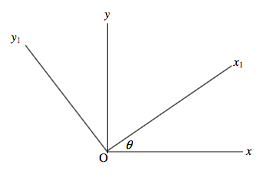$$\require{cancel}$$

2.12: Rotation of Axes

•• Contributed by Jeremy Tatum
• Emeritus Professor (Physics & Astronomy) at University of Victoria

We start by recalling a result from elementary geometry. Consider two sets of axes O$$xy$$ and O$$x_{1}y_{1}$$, the latter being inclined at an angle $$\theta$$ to the former. Any point in the plane can be described by the coordinates $$(x , y)$$ or by $$(x_{1} , y_{1})$$.These coordinates are related by a rotation matrix:

$\left(\begin{array}{c}x_{1}\\ y_{1}\end{array}\right) = \left(\begin{array}{c}\cos \theta \quad \sin \theta \\ -\sin \theta \quad \cos\theta\end{array}\right)\left(\begin{array}{c}x\\ y\end{array}\right), \label{eq:2.12.1}$

$\left(\begin{array}{c}x\\ y\end{array}\right) = \left(\begin{array}{c}\cos \theta \quad -\sin \theta \\ \sin \theta \quad \cos\theta\end{array}\right)\left(\begin{array}{c}x_{1}\\ y_{1}\end{array}\right). \label{eq:2.12.2}$

The rotation matrix is orthogonal; one of the several properties of an orthogonal matrix is that its reciprocal is its transpose.

Now let us apply this to the moments of inertia of a plane lamina. Let us suppose that the axes are in the plane of the lamina and that O is the centre of mass of the lamina. $$A, B$$ and $$H$$ are the moments of inertia with respect to the axes O$$xy$$ , and $$A_{1} , B_{1}$$ and $$H_{1}$$ are the moments of inertia with respect to O$$x_{1}y_{1}$$. Strictly speaking a lamina implies a continuous distribution of matter in a plane, but, since matter, we are told, is composed of discrete atoms, there is little difficulty in justifying treating a lamina as though it we a distribution of point masses in the plane. In any case the results that follow are valid either for a collection of point masses in a plane or for a genuine continuous lamina.

We have, by definition:

$A_{1} = \sum my^{2}_{1} \label{eq:2.12.3}$

$B_{1} = \sum mx^{2}_{1} \label{eq:2.12.4}$

$H_{1} = \sum mx_{1}y_{1} \label{eq:2.12.5}$

Now let us apply Equation $$\ref{eq:2.12.1}$$ to Equation $$\ref{eq:2.12.3}$$:

$$A_{1} = \sum m (-x \sin \theta + y \cos\theta )^2 = \sin^2 \theta \sum mx^2 - 2\sin\theta \cos \theta \sum mxy + \cos^2 \theta \sum my^2.$$

That is to say (writing the third term first, and the first term last)

$A_{1} = A \cos^2\theta -2H \sin \theta \cos \theta + B\sin^2 \theta. \label{eq:2.12.6}$

In a similar fashion, we obtain for the other two moments

$B_{1} = A \sin^2\theta +2H \sin \theta \cos \theta + B\cos^2 \theta. \label{eq:2.12.7}$

and

$H_{1} = A \sin\theta \cos \theta + H \sin(\cos^2 \theta - \sin^2 \theta) - B\sin\theta \cos \theta. \label{eq:2.12.8}$

It is usually more convenient to make use of trigonometric identities to write these as

$A_{1} = \frac{1}{2} (B+ A) - \frac{1}{2}(B-A)\cos2\theta - H \sin 2 \theta, \tag{2.12.9}\label{eq:2.12.9}$

$B_{1} = \frac{1}{2} (B+ A) + \frac{1}{2}(B-A)\cos2\theta + H \sin 2 \theta, \tag{2.12.10}\label{eq:2.12.10}$

$H_{1} = H \cos 2 \theta - \frac{1}{2}(B-A)\sin2 \theta \tag{2.12.11}\label{eq:2.12.11}$

These equations enable us to calculate the moments of inertia with respect to the axes O$$x_{1}y_{1}$$ if we know the moments with respect to the axes O$$xy$$. Further, a matter of importance, we see, from equation 2.12.11, that if

$\tan 2 \theta = \frac{2H}{B-A} , \label{eq:2.12.12}$

the product moment $$H_{1}$$ with respect to the axes $$Oxy$$ is zero. This gives some physical meaning to the product moment, namely: If we can find some axes (which we can, by means of Equation $$\ref{eq:2.12.12}$$) with respect to which the product moment is zero, these axes are called the principal axes of the lamina, and the moments of inertia with respect to the principal axes are called the principal moments of inertia. I shall use the symbols $$A_{0}$$ and $$B_{0}$$ for the principal moments of inertia, and I shall adopt the convention that $$A_{0} ≤ B_{0}$$.

Example $$\PageIndex{1}$$

Consider three point masses at the coordinates given below:

Mass Coordinates
5 (1 , 1)
3 (4 , 2 )
2 (3 , 4)

The moments of inertia are $$A = 49, B = 71, C = 53$$. The coordinates of the centre of mass are (2.3 , 1.9). If we use the parallel axes theorem, we can find the moments of inertia with respect to axes parallel to the original ones but with origin at the centre of mass. With respect to these axes we find $$A = 12.9, B = 18.1, H = +9.3$$. The principal axes are therefore inclined at angles $$\theta$$ to the $$x$$ -axis given (Equation $$\ref{eq:2.12.12}$$) by $$\tan 2 \theta = 3.57669$$; That is $$\theta$$ = 37°11' and 127° 11'. On using Equation $$\ref{eq:2.12.9}$$ or 10 with these two angles, together with the convention that $$A_{0} ≤ B_{0}$$ , we obtain for the principal moments of inertia $$A_{0} = 5.84$$ and $$B_{0} = 25.16$$.

Example $$\PageIndex{2}$$

Consider the right-angled triangular lamina of Section 11. The moments of inertia with respect to axes passing through the centre of mass and parallel to the orthogonal sides of the triangle are $$A= \frac{1}{18} Mb^ 2, B= \frac{1}{18} Ma^2, H=− \frac {1}{36} Mab$$. The angles that the principal axes make with the $$a$$- side are given by $$\tan 2 \theta = \frac{ab}{b^2-a^2}$$ . The interested reader will be able to work out expressions, in terms of $$M, a, b,$$ for the principal moments.